# Geometric approximation

geometrical-optics approximation, ray approximation

A series of the form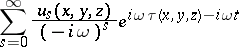that formally satisfies the equation describing a wave phenomenon (or a system of equations when theare vectors).

In order to solve wave propagation problems in the high-frequency regime (cf. Diffraction, mathematical theory of) the so-called ray method for the construction of geometric approximations has been developed , . Presumably, the resulting series is an asymptotic expansion of the solutions sought wherever the terms of the geometric approximation have no singular points. This hypothesis can be proved in special cases. There also exists a non-stationary analogue of the geometric approximation.

The construction of the functionsis based on considering the field of rays, i.e. the extremals of a functional (cf. Fermat principle):where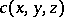is the wave speed in the isotropic physical medium under consideration and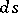is the element of arc length. Let some pair of parameters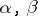characterize the ray, let the parametercharacterize the points on the ray, and letThe parameters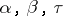may be used as curvilinear coordinates. The transition from these coordinates to the orthogonal Cartesian coordinate system is given by the formulaThe surfacesare orthogonal to the rays. At the points where the field of rays has no singularities, the magnitudewhich is known as the geometric spreading or divergence, is non-zero. The magnitude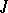forms part of recurrence relations interconnecting the functions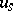, and plays a fundamental role in all constructions of geometric approximations.